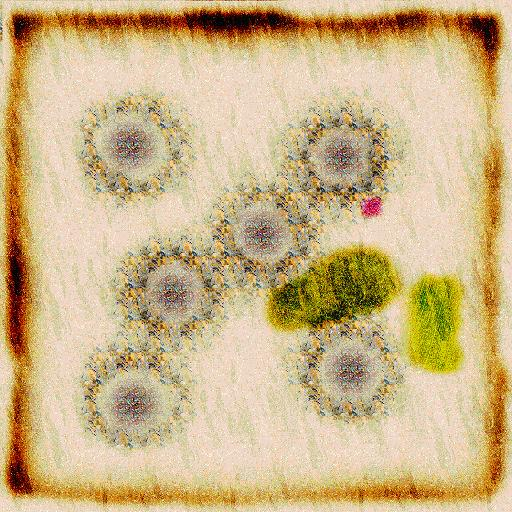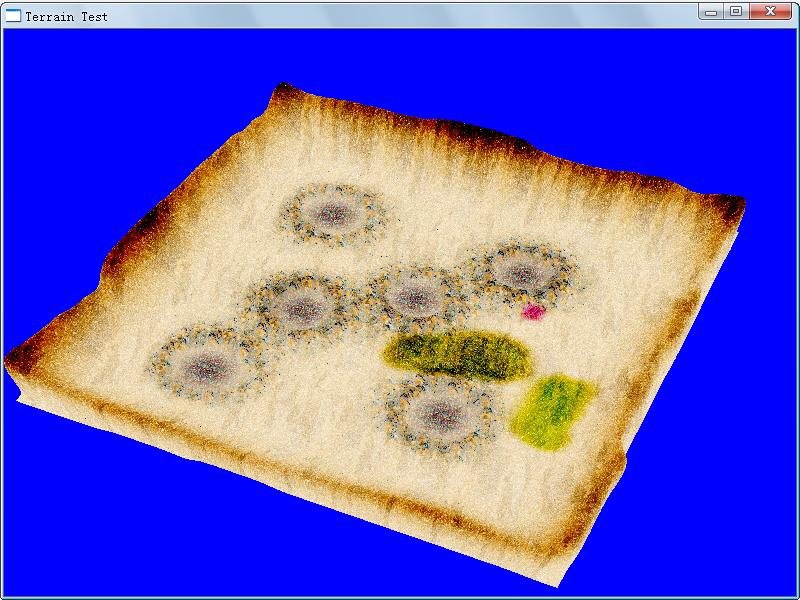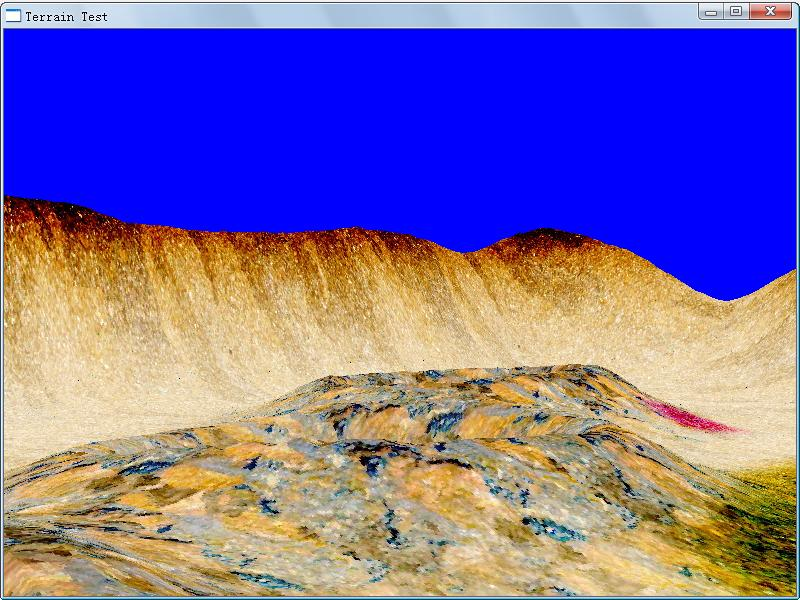## Direct3D学习（五）：基于高度图的地形生成

为什么80%的码农都做不了架构师？->>>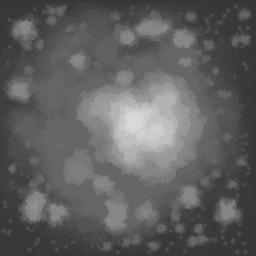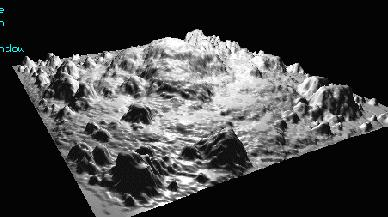x = pixel.x – image.width/2
z = -(pixel.y – image.height/2)
y = pixel.value/10          //因为255太大，需要适当缩小一下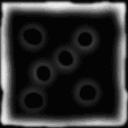绘制出来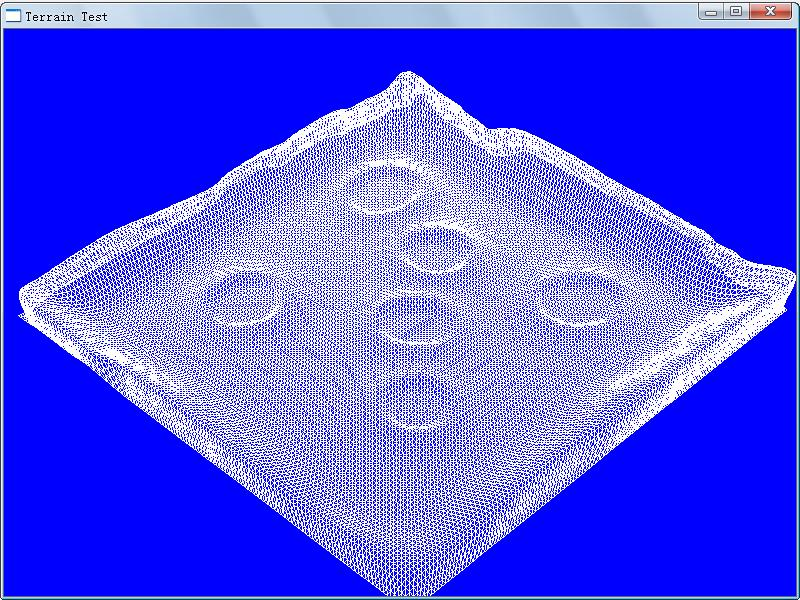for(int x = 0; x < GetWidth() - 1; x++)      {          for(int z = 0; z < GetHeight() - 1; z++)          {               index._0 = x * GetHeight() + z;               index._1 = x * GetHeight() + z + 1;               index._2 = (x + 1) * GetHeight() + z;               *pIndex++ = index;               index._0 = (x + 1) * GetHeight() + z;               index._1 = x * GetHeight() + z + 1;               index._2 = (x + 1) * GetHeight() + z + 1;               *pIndex++ = index;          }      }